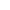Chapter 12 : Heron's Formula
Q. The triangular side walls of a flyover have been used for advertisements. The sides of the walls are 122 m, 22 m and 120 m (see figure). The advertisements yield an earning of Rs.5000 per m2 per year. A company hired one of its walls for 3 months. How much rent did it pay?View SolutionQ. There is a slide on a park. One of its side walls has been painted in some colour with a message "KEEP THE PARK GREEN AND CLEAN" (see figure). If the sides of the wall are 15 m, 11 m and 6 m, find the area painted in colour.View SolutionQ. A traffic signal board, indicating SCHOOL AHEAD, is an equivalent triangle with side 'a'. Find the area of the signal board, using Heron's formula. If its perimeter is 180 cm, what will be the area of the signal board?
View SolutionQ. Find the area of a triangle two sides of which are 18 cm and 10 cm and the perimeter is 42 cm.
View SolutionQ. Sides of a triangle are in the ratio of 12:17:25 and its perimeter is 540 cm. Find its area.
View SolutionQ. An isosceles triangle has perimeter 30cm and each of the equal sides is 12cm. Find the area of the triangle.
View SolutionQ. Find the area of a quadrilateral ABCD in which AB=3 cm, BC=4 cm, CD=4 cm, DA=5 cm and AC=5 cm
View SolutionQ. A rhombus shaped field has green grass for 18 cows to graze. If each side of the rhombus is 30 m and its longer diagonal is 48 m, how much area of grass field will each cow be getting?
View SolutionQ. A park, in the shape of a quadrilateral ABCD, has C=90o, AB=9 m, BC=12 m, CD=5 m and AD=8 m. How much area does it occupy?
View SolutionQ. A field is in the shape of a trapezium whose parallel sides are 25 m and 10 m. The non-parallel sides are 14 m and 13 m. Find the area of the field.
View SolutionQ. A floral design on a floor is made up of 16 tiles which are triangular, the sides of the triangle being 9 cm, 28 cm and 35 cm (see figure). Find the cost of polishing the tiles at the rate of 50 p per cm2.View SolutionQ. Radha made a picture of an aeroplane with coloured paper as shown in Fig 12.15. Find the total area of the paper used.View SolutionQ. An umbrella is made by stitching 10 triangular pieces of cloth of two different colours (see figure), each piece measuring 20 cm, 50 cm and 50 cm. How much cloth of each colour is required for the umbrella?View SolutionQ. A kite in the shape of square with a diagonal 32cm and an isosceles triangle of base 8cm and sides 6cm each is to be made of three different shades as shown in figure. How much paper if each shade has been used in it?View SolutionQ. A triangle and a parallelogram have the same base and the same area. If the sides of the triangle are 26cm, 28cm and 30cm and the parallelogram stands on the base 28cm, find the height of the paraollelogram.
View Solution